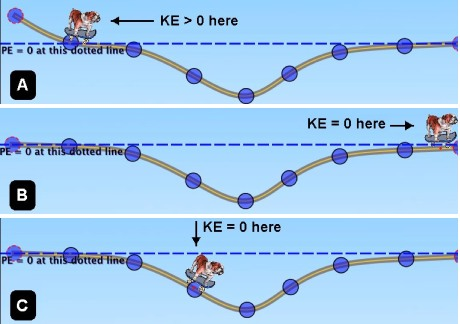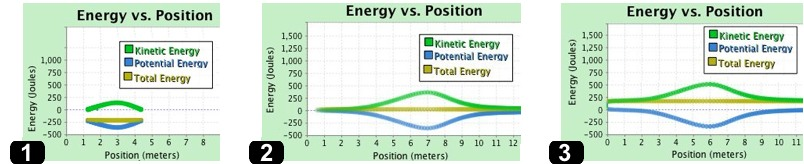Teaching Physics with the Physics Suite Edward F. Redish Problems Sorted by Type | Problems Sorted by Subject | Problems Sorted by Chapter in UP

The bulldog on the skateboard

 In the three figures labeled A, B, and C at the right are shown three situations in which a bulldog on a skateboard traverses a dip in the ground. (The dips are all the same.) In A, the bulldog enters the dip traveling at a non-0 kinetic energy (KE). In B, when he leaves the dip, he has 0 KE. In C, he begins in the dip (at the position indicated) at a KE of 0. Since he is riding a skateboard, friction may be ignored.A. In the figures below are shown graphs of the KE, gravitational PE, and total energy as a function of position. In situation 1, the total energy is negative; in situation 2, the total energy is 0; and in situation 3, the total energy is positive. Match which situation goes with which graph and put the letter of the situation to the left of the corresponding graphB. The bulldog and skateboard have a combined mass of 20 kg. In case B (the middle of the three pictures of the bulldog and the well), the bulldog and skateboard have a KE of 380 J when they roll past the bottom of the well. How deep is the well?

C. In case 1 (the left one of the three graphs) it says the KE is positive but the total energy is negative. How is this possible? Explain what it means for the total energy to be negative.

D. In case 3, the total energy is 200 J. How fast is the bulldog when he passes the bottom of the well?